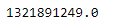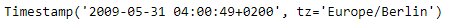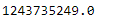# Python | Pandas Timestamp.timestamp

• Last Updated : 21 Jan, 2019

Python is a great language for doing data analysis, primarily because of the fantastic ecosystem of data-centric python packages. Pandas is one of those packages and makes importing and analyzing data much easier.

Pandas` Timestamp.timestamp()` function return the time expressed as the number of seconds that have passed since January 1, 1970. That zero moment is known as the epoch.

Attention geek! Strengthen your foundations with the Python Programming Foundation Course and learn the basics.

To begin with, your interview preparations Enhance your Data Structures concepts with the Python DS Course. And to begin with your Machine Learning Journey, join the Machine Learning - Basic Level Course

Syntax :Timestamp.timestamp()

Parameters : None

Return : number of seconds since zero moment

Example #1: Use `Timestamp.timestamp()` function to return the number of seconds that has passed since the zero moment for the given Timestamp object.

 `# importing pandas as pd``import` `pandas as pd`` ` `# Create the Timestamp object``ts ``=` `pd.Timestamp(year ``=` `2011``,  month ``=` `11``, day ``=` `21``, ``                  ``hour ``=` `10``, second ``=` `49``, tz ``=` `'US/Central'``) `` ` `# Print the Timestamp object``print``(ts)`

Output :Now we will use the `Timestamp.timestamp()` function to find the number of seconds that has passed.

 `# return the number of seconds``ts.timestamp()`

Output :As we can see in the output, the `Timestamp.timestamp()` function has returned a float value indicating this many seconds has passed since the epoch for the given Timestamp object.

Example #2: Use `Timestamp.timestamp()` function to return the number of seconds that has passed since the zero moment for the given Timestamp object.

 `# importing pandas as pd``import` `pandas as pd`` ` `# Create the Timestamp object``ts ``=` `pd.Timestamp(year ``=` `2009``, month ``=` `5``, day ``=` `31``, ``                  ``hour ``=` `4``, second ``=` `49``, tz ``=` `'Europe/Berlin'``)`` ` `# Print the Timestamp object``print``(ts)`

Output :Now we will use the `Timestamp.timestamp()` function to find the number of seconds that has passed.

 `# return the number of seconds``ts.timestamp()`

Output :As we can see in the output, the `Timestamp.timestamp()` function has returned a float value indicating this many seconds has passed since the epoch for the given Timestamp object.

My Personal Notes arrow_drop_up